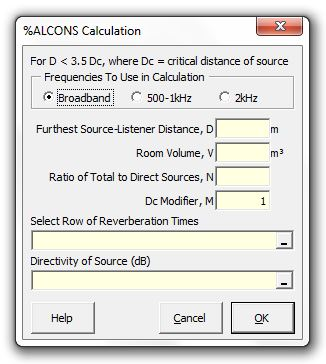### Strutt Help

%ALCONS Calculation    1/1,1/3

Strutt|Electroacoustics|%ALcons calculates the articulation loss of consonants as a percentage and inserts the result into the active row of the work sheet.Strutt implements the formula as:

%AL_(cons) = (200 \ D_2^2 \ RT^2 \ N_(t ot))/(VQMN_(dir))

where,
RT is the reverberation time, s
V is the room volume, m3
N_(t ot) is the total number of loud speakers
N_(dir) is the number of loudspeaker contributing to direct (early) sound
Q is the directivity factor of source
M is a specialist modifier (assume M=1 for most situations)
D_2 is the furthest distance from loudspeaker, m